# Current Electricity

### Guest Account

Attempt Form Three Physics Questions
Guest Account

# Current Electricity

## Electric potential difference and electric current

#### Electric current

- Electric potential difference (p. d) is defined as the work done per unit charge in moving charge from one point to another. It is measured in volts.
- Electric current is the rate of flow of charge. P. d is measured using a voltmeter while current is measured using an ammeter. The SI units for charge is amperes (A).

#### Ammeters and voltmeters

- In a circuit an ammeter is always connected in series with the battery while a voltmeter is always connected parallel to the device whose voltage is being measured.

#### Ohm’s law

- This law gives the relationship between the voltage across a conductor and the current flowing through it. Ohm’s law states that the current flowing through a metal conductor is directly proportional to the potential difference across the ends of the wire provided that temperature and other physical conditions remain constant.
Mathematically V ∝ I
So V/I = constant, this constant of proportionality is called resistance
V/I = Resistance (R)
Resistance is measured in ohms and given the symbol Ω

Example 1
A current of 2mA flows through a conductor of resistance 2 kΩ. Calculate the voltage across the conductor.
Solution

V = IR = (2 × 10-3) × (2 × 103) = 4 V.

Example 2
A wire of resistance 20Ω is connected across a battery of 5 V. What current is flowing in the circuit?
Solution

I = V/R = 5 / 20 = 0.25 A

#### Ohmic and non-ohmic conductors

Ohmic conductors are those that obey Ohms law (V ∝ I) and a good example is nichrome wire i.e. the nichrome wire is not affected by temperature.
Non-ohmic conductors do not obey Ohms law i.e. bulb filament (tungsten), thermistor couple, semi-conductor diode etc. They are affected by temperature hence non-linear.

Factors affecting the resistance of a metallic conductor
1. Temperature – resistance increases with increase in temperature.
2. Length of the conductor – increase in length increases resistance.
3. Cross-sectional area – resistance is inversely proportional to the cross-sectional area of a conductor of the same material.

#### Resistivity

The Resistivity of a material is numerically equal to the resistance of a material of unit length and unit cross-sectional area. It is symbolized by ρ and the units are ohmmeter (Ωm). It is given by the following formula;
ρ = AR /l where A – cross-sectional area, R – resistance, l – length

Example 3
Given that the resistivity of nichrome is 1.1× 10-6 Ωm, what length of nichrome wire of diameter 0.42 mm is needed to make a resistance of 20 Ω?
Solution

ρ = AR /l, hence l = RA/ ρ = 20 × 3.142 × (2.1 × 10 - 4) / 1.1 × 10 - 6 = 2.52 m

## Resistors

Resistors are used to regulate or control the magnitude of current and voltage in a circuit according to Ohms law.

#### Types of resistors

i) Fixed resistors – they are wire-wound or carbon resistors and are designed to give a fixed resistance.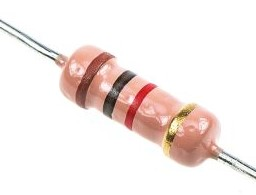ii) Variable resistors – they consist of the rheostat and potentiometer. The resistance can be varied by sliding a metal contact to generate desirable resistance.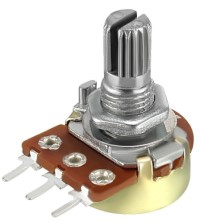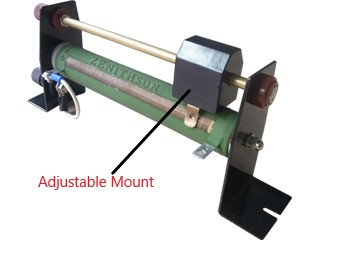#### Resistor combination

a) Series combination
Consider the following loop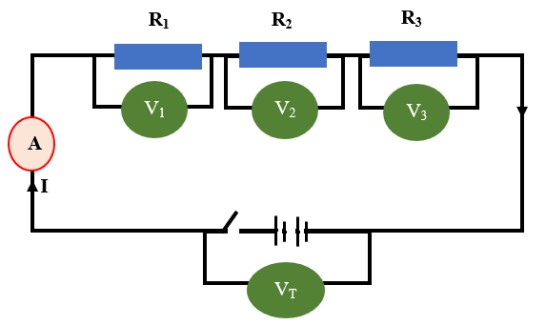- Since it is in series then,
VT = V1 + V2 + V3
The same current (I) flows through the circuit (resistors), hence
IRT = I (R1 + R2 + R3), dividing through by I, then
RT = R1 + R2 + R3
Therefore for resistors connected in series the equivalent resistance (Req) is equal to the total sum of their individual resistances.
Req = R1 + R2 + R3

b) Parallel combination
Consider the following circuit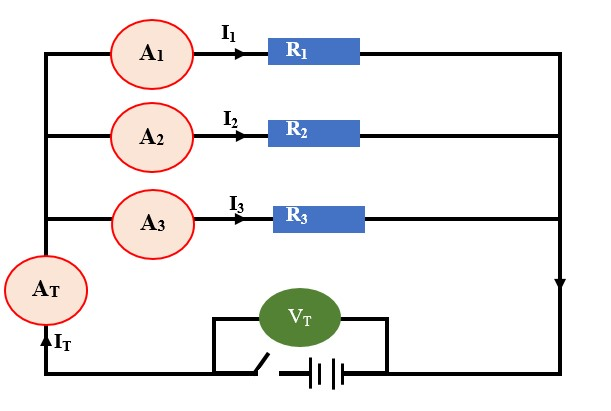Total current is given by:
IT = I1 + I2 + I3. But IT = VT/RT = V1/R1 + V2/R2 + V3/R3
Since in parallel, VT = V1 = V2 = V3
Then 1/RT = 1/R1 + 1/R2 +1/R3, for 'n' resistors in parallel
1/RT = 1/R1 + 1/R2 +1/R3 ………… 1/R n
If only two resistors are involved then the equivalent resistance becomes
1/Req = 1/R1 + 1/R2 = (R1 + R2)/ R1 R2

Example 4
1. Calculate the effective resistance in the following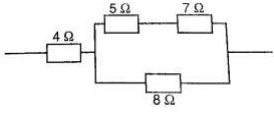Solution

This reduces to: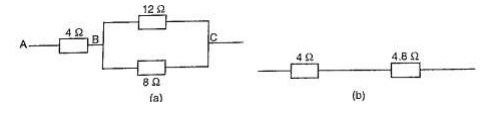Combining the two in parallel;
1/Req = (R1 + R2)/R1 R1 = 20/96
1/Req = 20/96, therefore Req = 96/20 = 4.8 Ω
Lastly combining the two in series;
Then Req = 4 Ω + 4.8 Ω = 8.8 Ω

Example 5
In the diagram below, a current of 0.8 A, passing through an arrangement of resistors as shown below. Find the current through the 10 Ω resistor.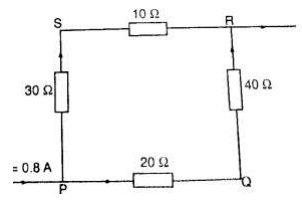Solution

Combining those in series then this can be replaced by two resistors of 60 Ω and 40 Ω.
Current through 10 Ω = (p.d. between P and R)/ (30 + 10) Ω
p.d between P and R = 0.8 × Req. Req = (40 × 60)/ 40 + 60 = 2400/ 100 = 24 Ω
p.d across R and P = 0.8 × 24 (V = IR)
therefore, current through 10 Ω = 19.2 / 10 + 30 = 0.48 A

#### Electromotive force and internal resistance

Electromotive force (e.m.f.) is the p.d across a cell when no current is being drawn from the cell.
Consider the following diagram;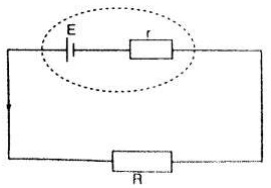The current flowing through the circuit is given by the equation,
Current = e.m.f / total resistance
I = E / R + r where E – e.m.f of the cell
Therefore E = I (R + r) = IR + I r = V + I r

Example 6
A cell drives a current of 0.6 A through a resistance of 2 Ω. if the value of resistance is increased to 7 Ω the current becomes 0.2 A. calculate the value of e.m.f of the cell and its internal resistance.
Solution

Let the internal resistance be 'r' and e.m.f be 'E'.
Using E = V + I r = IR + Ir
Substitute for the two sets of values for I and R
E = 0.6 × (2 + 0.6 r) = 1.2 + 0.36 r
E = 0.6 × (7 × 0.2 r) = 1.4 + 0.12 r
Solving the two simultaneously, we have,
E = 1.5 v and R = 0.5 Ω

Example 7
A battery consists of two identical cells, each of e.m.f 1.5 v and internal resistance of 0.6 Ω, connected in parallel. Calculate the current the battery drives through a 0.7 Ω resistor.
Solution

When two identical cells are connected in series, the equivalent e.m.f is equal to that of only one cell. The equivalent internal resistance is equal to that of two such resistance connected in parallel. Hence Req = R1 R2 / R1 + R2 = (0.6 × 0.6) / 0.6 + 0.6 = 0.36 / 1.2 = 0.3 Ω
Equivalent e.m.f =1.5 / (0.7 + 0.3) = 1.5 A
Hence current flowing through 0.7 Ω resistor is 1.5 A